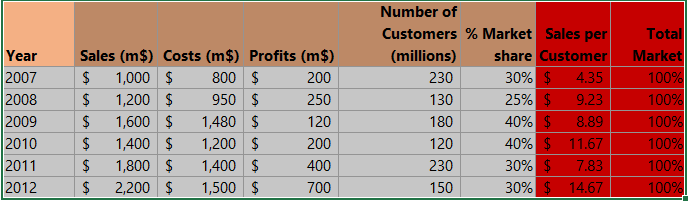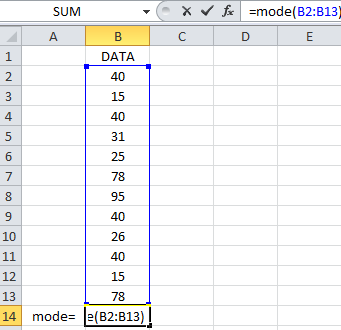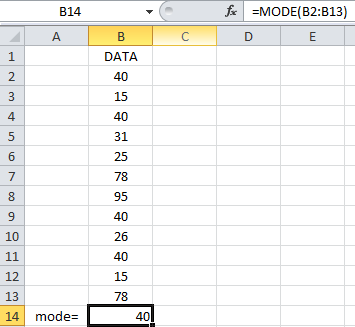#### How to calculate mode?

Mode is the most frequently occurring number in a given data set. To calculate the mode in Excel you need to use dedicated Excel function as I am trying to show you below.

To learn how to calculate the mode in Excel, follow the steps below. We are going to use dummy data.

## How to find the Mode?### Mode function

Mode function returns the most frequently occurring value in a range of cells. To use the MODE function, enter the following formula in the cell where you want to display the mode: “=MODE(range)”. Replace “range” with the range of cells that you want to find the mode of.

Put the formula =Mode(B2:B13) in the formula bar (fx).Press enter, and that’s it.The mode has now been calculated.

In the newer Excel versions (Excel 2021 and Excel 365), there are two new functions introduced. MODE.SNGL and MODE.MULT have been added. You should use them if you need a single-mode or multiple-mode display.

### MODE.MULT function

Mode.mult function returns the most frequently occurring values in a range of cells. To use the MODE.MULT function, enter the following formula in the cell where you want to display the mode: “=MODE.MULT(range)”. Replace “range” with the range of cells that you want to find the mode of.

Note: If there are multiple values with the same frequency, the MODE.MULT function will return all of those values.